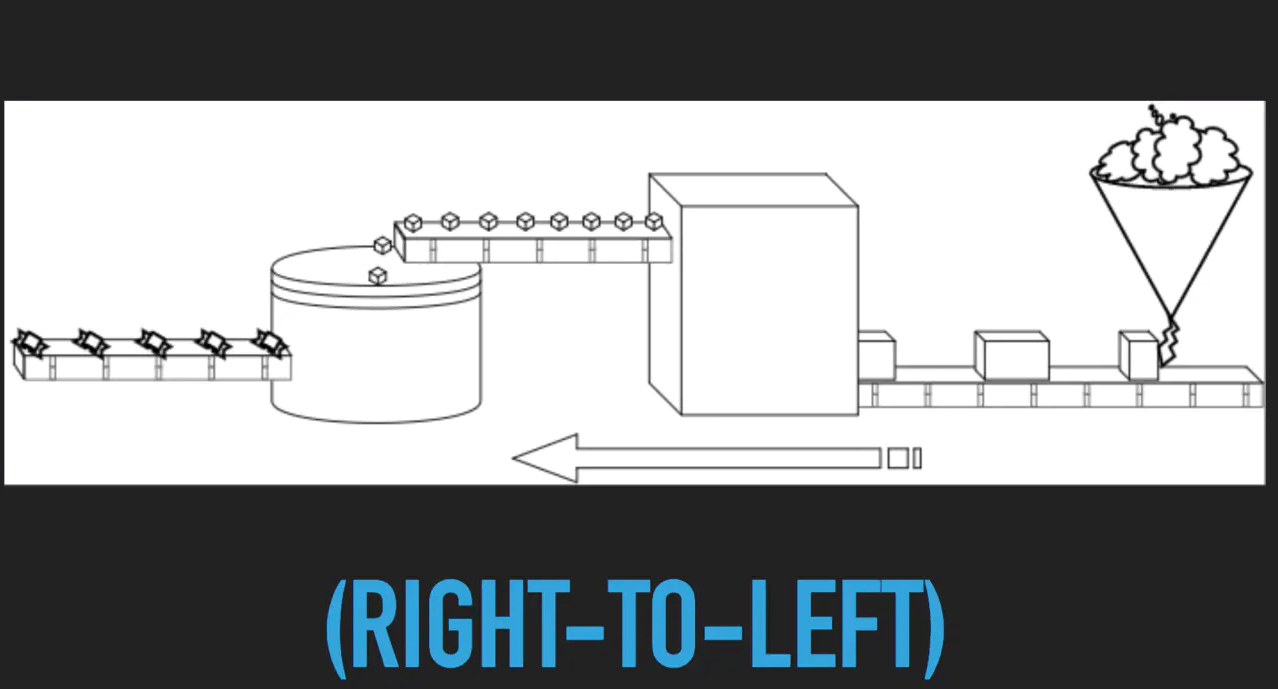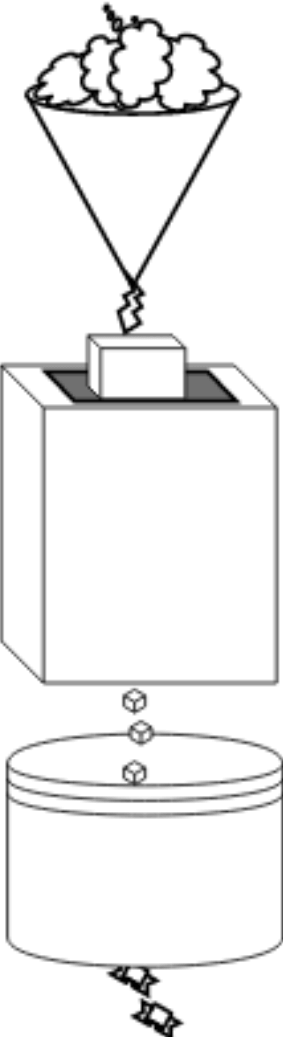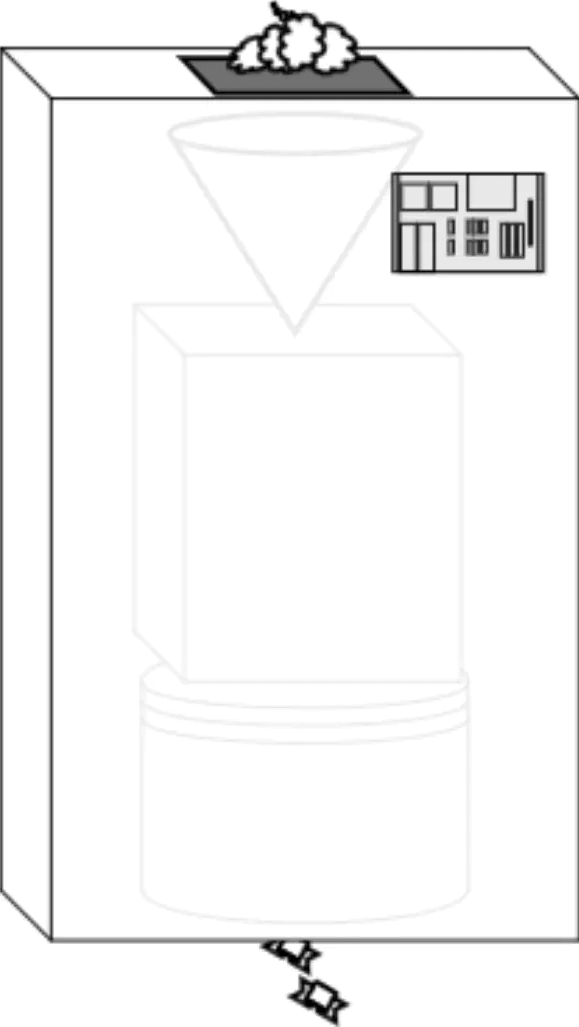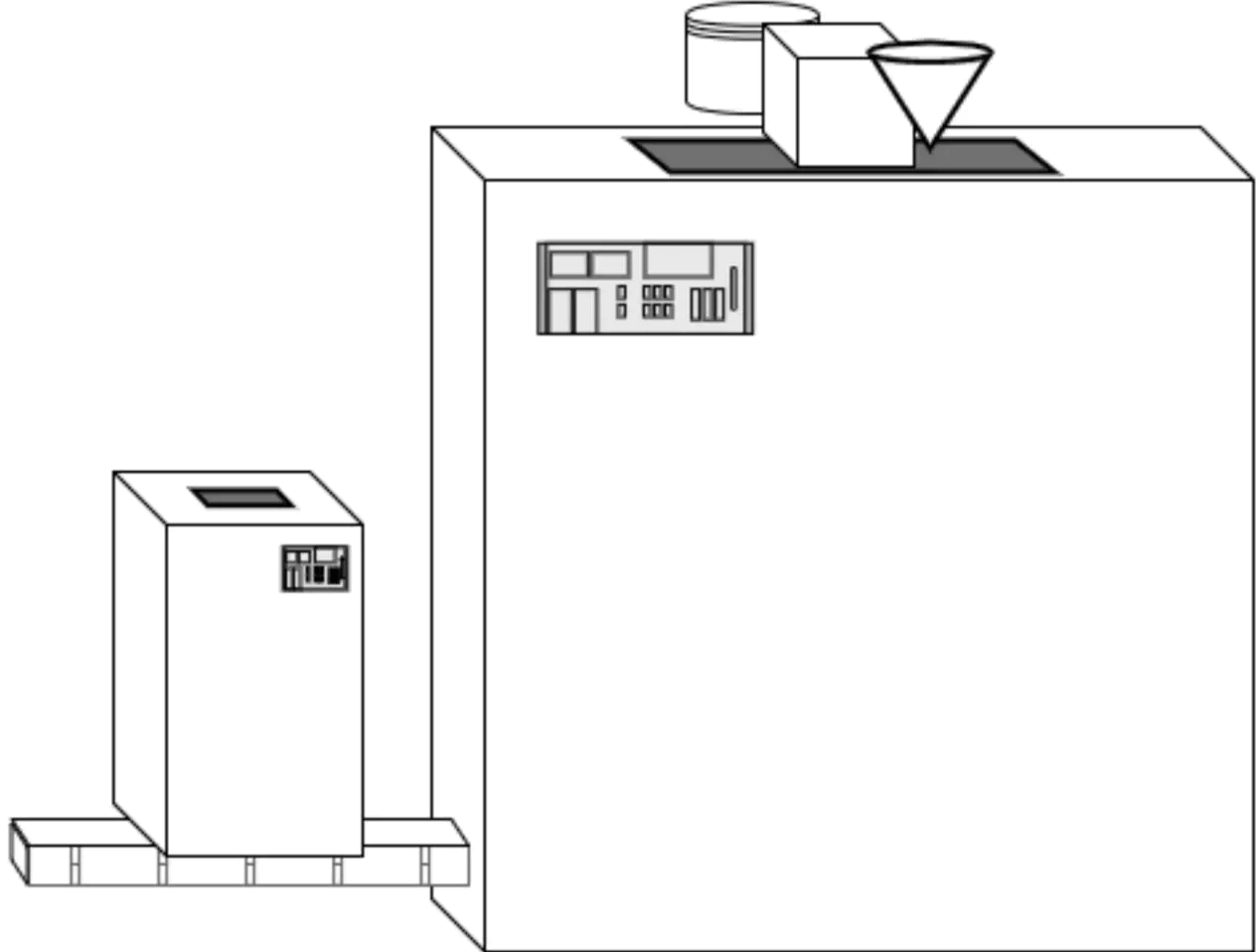# 函数式初识(6) - 组合

by Teobler on 03 / 09 / 2019

views

Point-free的文章中我们简单的提到过组合(compose)技术，现在我们来做一些比较沉入的了解。

``````function minus2(x) { return x - 2; }
function triple(x) { return x * 3; }
function increment(x) { return x + 1; }

// 算总价
let temp = increment(4);
temp = triple(temp);
totalCost = basePrice + minus2(temp);````````totalCost = basePrice + minus2(triple(increment(4)));````````function shippingRate(x) {
return minus2(triple(increment(x)));
}

totalCost = basePrice + shippingRate(4);````````````function composeThree(fn2, fn1, fn0) {
return function composed(v) {
return fn2(fn1(fn0(v)));
}
}``````

``````const shippingRate = composeThree(minus2, triple, increment);

totalCost = basePrice + shippingRate(4);``````

``````const internetShippingRate = composeThree(double, increment, minus1);

const xxxShippingRate = composeThree(increment, triple, minus4);``````### Pipe VS Compose

Compose是一种从右到左的定义方式，相对应的，Pipe是一种从左到右的定义方式。

``````function minus2(x) { return x - 2; }
function triple(x) { return x * 3; }
function increment(x) { return x + 1; }

const f = composeThree(minus2, triple, increment);
const p = pipeThree(increment, triple, minus2);

f(4); // 7
g(4); // 7``````

### 结合律与柯里化

``compose(fn1, compose(fn2, fn3)) === compose(compose(fn1, fn2), fn3);``

``````function sum(x, y) { return x + y; }
function triple(x) { return x * 3; }
function divBy(y, x) { return x / y; }

divBy(2, triple(sum(3, 5))); // 12

sum = curry(2, sum);
divBy = curry(2, divBy);

compose(
divBy(2),
triple,
sum(3)
)(5);
// 12``````

### Point-free with composition

``````const mod2 = mod(2);
const eq1 = eq(1);

function isOdd(x) {
return eq1(mod(x));
}

const isOdd = compose(eq1, mod2);
const isOdd = compose(eq(1), mod(2));``````